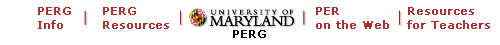University of Maryland Physics Education Research GroupUMD PERG PhD Dissertations: Eric Kuo

PERG Info | PERG materials | PERG HOMEPAGE | PER on the web | Resources on the web

More than just "plug-and-chug": Exploring how physics students make sense with equations

Abstract

Although a large part the Physics Education Research (PER) literature investigates students’ conceptual understanding in physics, these investigations focus on qualitative, conceptual reasoning. Even in modeling expert problem solving, attention to conceptual understanding means a focus on initial qualitative analysis of the problem; the equations are typically conceived of as tools for “plug-and-chug” calculations. In this dissertation, I explore the ways that undergraduate physics students make conceptual sense of physics equations and the factors that support this type of reasoning through three separate studies.

In the first study, I investigate how students’ can understand physics equations intuitively through use of a particular class of cognitive elements, symbolic forms (Sherin, 2001). Additionally, I show how students leverage this intuitive, conceptual meaning of equations in problem solving. By doing so, these students avoid algorithmic manipulations, instead using a heuristic approach that leverages the equation in a conceptual argument.

The second study asks the question why some students use symbolic forms and others don’t. Although it is possible that students simply lack the knowledge required, I argue that this is not the only explanation. Rather, symbolic forms use is connected to particular epistemological stances, in-the-moment views on what kinds of knowledge and reasoning are appropriate in physics. Specifically, stances that value coherence between formal, mathematical knowledge and intuitive, conceptual knowledge are likely to support symbolic forms use. Through the case study of one student, I argue that both reasoning with equations and epistemological stances are dynamic, and that shifts in epistemological stance can produce shifts in whether symbolic forms are used to reason with equations.

The third study expands the focus to what influences how students reason with equations across disciplinary problem contexts. In seeking to understand differences in how the same student reasons on two similar problems in calculus and physics, I show two factors, beyond the content or structure of the problems, that can help explain why reasoning on these two problems would be so different. This contributes to an understanding of what can support or impede transfer of content knowledge across disciplinary boundaries.

• I. Introduction and Literature Review
• II. Theoretical Framework
• III. How Students Blend Conceptual and Formal Mathematical Reasoning in Solving Physics Problems
• IV. Connecting Epistemology to Symbolic Forms Use
• V. Looking Beyond Content and Structure to Understand Transfer of Mathematical Approaches Across Disciplinary Contexts
• VI. Summary and Future Directions

Back to UMD PERG PhD DissertationsMaintained by University of Maryland PERG
Com8ments and questions may be directed to E. F. Redish